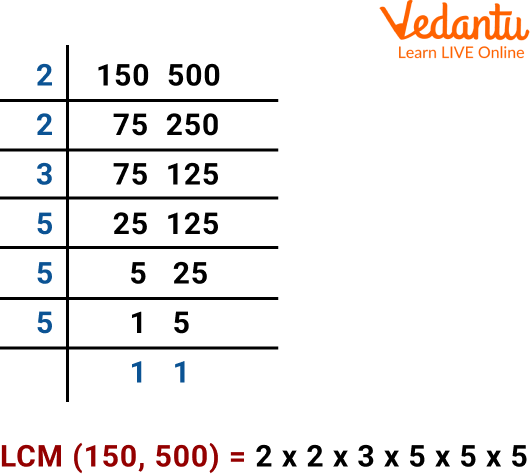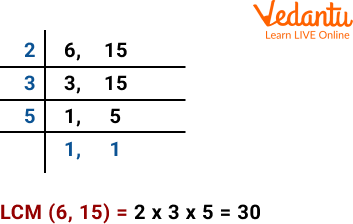Courses
Courses for Kids
Free study material
Free LIVE classes
More

# LCM of Decimal Numbers## An Overview of LCM

The least Common Multiple is the meaning of the abbreviation LCM. The lowest number that may be divided by both numbers is the least common multiple (LCM) of two numbers. It can also be computed using two or more numbers. Finding the LCM of a given set of numbers can be done in various ways. Utilizing the prime factorization of each number and then calculating the product of the highest powers of the shared prime factors is one of the quickest techniques to determine the LCM of two numbers. In this article, we will learn how the lcm of decimal numbers can be found and see LCM of fractions formula.

## How to Find the LCM of Decimal Numbers?

To find the LCM of decimal numbers, we have two different methods. Anyone willing to use any of these can use it anywhere to solve LCM of decimal numbers. The two different methods to determine the LCM of a set of numbers are the following:

LCM by Using the Listing Method
In this method, we shift the decimal places before taking out the LCM and in the end, after doing prime factorization. We again move the decimal places back to the position.

Let’s understand it with an example:

LCM of 1.50 and 5.00 by Listing Method: For 1.50 and 5.00, move the decimal 2 places to the right. Now, they are whole numbers. We will find the LCM of 150 and 500. In the end, we will move the decimal point back to 2 places to the left.

Multiples of 150 are 150, 300, 450, 600, 750, 900, 1050, 1200, 1350, 1500, 1650, and 1800, ….

Multiples of 500 are 500, 1000, 1500, 2000, 2500, …

The lowest common multiple of 150 and 500 is 1500

So, LCM(150, 500) = 1500.

Now, we move the decimal towards 2 places to the left.Therefore LCM(1.50,5.00) = 15.00.

LCM by Using the Division Method

Let’s understand the LCM by using the division method through an example:

LCM of 1.50 and 5.00 by Common Division Method

For each of the numbers, we move the decimal to two places to the right and calculate the LCM of whole numbers 150 and 500. Then, in the end, we move the decimal to two places back.LCM of 150 and 500 is1500

We see that LCM of 150 and 500 is 1500. Since we multiplied by 100 to remove the decimal point, we will divide by 100, moving the decimal point two places back. So, we get the LCM of 1.5 and 5 as 15.

Hence, LCM of 1.50 and 5.00 is 15.

## LCM of Fractions Formula

To solve any problem efficiently, one must know its formula. In the same way, finding out the LCM of fractions requires some formula. To find L.C.M of $\dfrac{a}{b}$ and $\dfrac{c}{d}$ the generalized formula will be:

L.C.M $=\dfrac{\text { L.C.M of numerators }}{\text { H.C.F of denominators }}$.

Now L.C.M of two numbers is the smallest number (not zero), a multiple of both.

Let's take the example of $\dfrac{2}{5} \text { and } \dfrac{3}{7}$

$\text { L.C.M }=\dfrac{\text { L.C.M of }(2,3)}{\text { H.C.F of }(5,7)}$ ....(1)

So H.C.F of 5,7:

The factors of 5 are: 1,5

The factors of 7 are: 1,7

1 is the only common factor as it is the only number common to both 5 and 7.

Therefore, H.C.F of $(5,7)=1$. .....(2)

Now L.C.M of 2, 3:

The multiples of 2 are $2,4,6,8, \ldots$.

The multiples of 3 are $3,6,9,12, \ldots$

6 is the lowest common multiple as it is a multiple common to both 2 and 3.

Therefore, L.C.M of $(2,3)=6$ .......(3)

Putting the value of H.C.F from equation (2) and the value of L.C.M from equation (3) in equation (1), we get L.C.M $=\dfrac{6}{1}=6$

LCM calculator with work can be done by using the online calculator, which is available on the internet. Through this calculator, we can easily find the LCM online.

## Solved Examples

Q 1. Find the least common multiple (LCM) of 6 and 15 using the division method.

Ans: Let us find the least common multiple (LCM) of 6 and 15 using the division method using the steps given below.

Step 1: 2 is the smallest prime number, a factor of 6. Write 2 on the left of the two numbers. For each number in the right column, continue finding prime numbers and their factors.

Step 2: 2 divides 6, but it is not a factor of 15, so we write the number 15 in the row below as it is. Continue the steps until 1 is left in the last row. Then, we divide 3 and 15 by 3. This gives us 1 and 3. We write 5 on the left side and finally get 1, 1 as the quotient in the last row.

Step 3: Then, we multiply these numbers on the left. The LCM is the product of all these prime numbers. LCM of 6 and 15 is 2 × 3 × 5 = 30.LCM of 6 and 15

Q 2. Find the LCM of 25, 15, and 30 by listing method.

Ans: Let us use the following steps to find the LCM of the 3 numbers.

Step 1: List the first few multiples of all three numbers, This will be:

Multiples of 25 = 25, 50, 75, 100, 125, 150, 175, ....,

Multiples of 15 = 15, 30, 45, 60, 75, 90, 105, 120, 135, 150, 175,...

Multiples of 30 = 30, 60, 90, 120, 150, 180, 210, ...

Step 2: Among the common multiples of 25, 15 and 30, we can see that 150 is the least multiple that is common in all three numbers.

Therefore, the LCM of 25, 15 and 30 = 150.

## Practice Questions

Q 1. Find the LCM of 0.48, 0.72, and 0.108.

Ans: 4.32

Q 2. Find the LCM of $\dfrac{1}{3}$, $\dfrac{1}{6}$, and $\dfrac{1}{9}$.

Ans: $\dfrac{1}{18}$

Q 3. Calculate the LCM of 0.8, 0.2, and 4.8.

Ans: 4.8

## Summary

Finding out LCM is quite easy to learn and remember. Interestingly, the division method is highly used to find the LCM of any two or three numbers. We are sure the kids have learned in depth about the LCM of decimal numbers, how to find the LCM of decimal numbers, the LCM of fractions, the LCM calculator with work, and many more. Other than this, solving various types of practice questions will help the kids master the particular topic in a better way.

Last updated date: 22nd May 2023
Total views: 40.2k
Views today: 1.22k

## FAQs on LCM of Decimal Numbers

1. Who first invented and used LCM in the mathematical world?

The first person to invent LCM was the famous mathematician Euclid. LCM was first used way back in 325-270 BC.

2. Why is solving LCM very important?

Learning and solving LCM problems are quite important as it helps in the thought process. Besides that, it plays a significant role in adding, subtracting, and comparing two or more fractions.

3. Is LCM used in accounting?

Fortunately, LCM is used in accounting. It is used when an investor value a company’s inventory, those assets are recorded on the balance sheet.